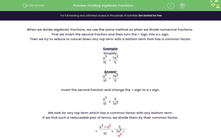# Dividing Algebraic Fractions

In this worksheet, students divide algebraic fractions giving their answers as a single fraction.Key stage:  KS 3

Curriculum topic:   Algebra

Curriculum subtopic:   Use and Interpret Algebraic Notation

Difficulty level:#### Worksheet Overview

When we divide algebraic fractions, we use the same method as when we divide numerical fractions.

First we invert the second fraction and then turn the ÷ sign into a x sign.

Then we try to reduce or cancel down any top term with a bottom term that has a common factor.

Example

Simplify:

 a3 ÷ 7a2 10 5

 a3 ÷ 7a2 10 5

Invert the second fraction and change the ÷ sign to a x sign.

 a3 x 5 10 7a2

We look for any top term which has a common factor with any bottom term.

If we find such a reducible pair of terms, we divide them by their common factor.

 = a3 ÷ a2 x 5 10 7a2 ÷ a2

 = a x 5 10 7

 = a x 5 ÷ 5 10 ÷ 5 7

 = a x 1 2 7

 = a 14

### What is EdPlace?

We're your National Curriculum aligned online education content provider helping each child succeed in English, maths and science from year 1 to GCSE. With an EdPlace account you’ll be able to track and measure progress, helping each child achieve their best. We build confidence and attainment by personalising each child’s learning at a level that suits them.

Get started